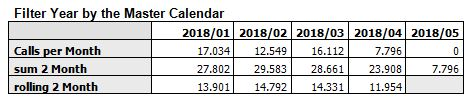# QlikView App Development

Discussion Board for collaboration related to QlikView App Development.

Contributor

## as of table and rolling n months

Hello,

I have a question about the use of an "as of table" and calculating rolling n month.

When I calculate rolling 2 month in a chart by using the "AsOfMonth" dimension,

I have to select the whished Year by the field "AsOfYear" to get the correct rolling month value for the first displayed month like in the example. By using Year from the Master Calendar the January is not rolling 2 Month.Due to the usability of the Application I have to use the Filter from the Master Calendar for this chart too.

Currently I use a expression like:  sum(  { < MonthDiff={"<2"}  > } [Calls] )

Is there a possibility to customize the expression that I can use the Master Calendar for Selections too?

Many thanks in advance and best regards,

Claus

Tags (3)
1 Solution

Accepted Solutions
Contributor

## Re: as of table and rolling n months

I think I get the solution...

By selection Year from my Master Calendar and using the below expression,

I get the whished result of the aggregation of the previous and the current month.

=aggr( above ( Sum( { 1 < MonthDiff={"<2"} > } [Calls]) , 0, 2 ), AsOfMonth )

And by using this expression I get a rolling two month average,

=avg( aggr( rangeavg( below( sum ({ 1 < MonthDiff={"<1"} > } [Calls]) , 0, 2 )), AsOfMonth ))There are still some questions but over all this is it.

4 Replies
Contributor

## Re: as of table and rolling n months

Not 100% sure I understand your question, but this might be worth considering.

I typcially use Autonumber() in my calendar to give each YYMM its own value in chronological order.

Of course you must ensure that your Calendar is also built in chrono order and see whether each YYMM has its own correct ascending numerical value.

In the case example you give above, Jan 2018 would have autonum = 1; Feb 2018 have autonum = 2, etc. This you do in the script itself using "Autoonumber([YYMM field name]) as YYMM_Num.

Then in Qlik object you can use this "NUM" value in set analysis. I initially did this to prevent issues on change of Year values, e.g. if "Jan" is selected, how does the Qlik object interpret previous month, i.e. Dec of previous year.

In set analysis, you then create multiple copies of expressions such as:

sum(  { < YYMM_Num={\$(=MAX(YYMM_Num))}, YYMM=  > } [Calls] )

sum(  { < YYMM_Num={\$(=MAX(YYMM_Num)-1)}, YYMM=  > } [Calls] )

sum(  { < YYMM_Num={\$(=MAX(YYMM_Num)-2)}, YYMM=  > } [Calls] )

etc.

I think you might be able to find some variant on your rolling 2 months and by-passing the actual date selection, espcially with greater-than and lesser-than in the set analysis.

Otherwise, please try and share an app with sample data. It would be easier to see what you are trying to achieve.

Cheerio.

Contributor

## Re: as of table and rolling n months

I was following the example from here: The As-Of Table

The result of the expression should in this case by for January 2018 the sum of Dec-2017 + Jan-2018.

It works already by selecting the whished Year by the Year from the AsOfYear field but not by selecting the Year from the Master Calendar.

I have also a numeric value for each month. But the target is to find a solution by using the as of table.

Many thanks and best regards,

Claus

Contributor

## Re: as of table and rolling n months

Thanks for the link to Henrik Cronstorm's post. It sounds like an interesting question and problem. I'll look into it and see if I can reproduce something, as am interested in finding a solution to this. If you do find a solution yourself, please share.

KR,

Cheenu

Contributor

## Re: as of table and rolling n months

I think I get the solution...

By selection Year from my Master Calendar and using the below expression,

I get the whished result of the aggregation of the previous and the current month.

=aggr( above ( Sum( { 1 < MonthDiff={"<2"} > } [Calls]) , 0, 2 ), AsOfMonth )

And by using this expression I get a rolling two month average,

=avg( aggr( rangeavg( below( sum ({ 1 < MonthDiff={"<1"} > } [Calls]) , 0, 2 )), AsOfMonth ))There are still some questions but over all this is it.